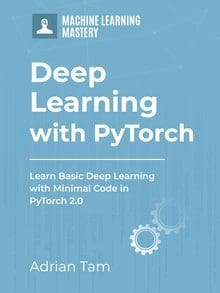# Training a Multi-Target Multilinear Regression Model in PyTorch

The multi-target multilinear regression model is a type of machine learning model that takes single or multiple features as input to make multiple predictions. In our earlier post, we discussed how to make simple predictions with multilinear regression and generate multiple outputs. Here we’ll build our model and train it on a dataset.

In this post, we’ll generate a dataset and define our model with an optimizer and a loss function. Then, we’ll train our model and visualize the results of the training process. Particularly, we’ll explain:

• How to train a multi-target multilinear regression model in PyTorch.
• How to generate a simple dataset and feed it to the model.
• How to build the model using built-in packages in PyTorch.
• How to train the model with mini-batch gradient descent and visualize the results.

Kick-start your project with my book Deep Learning with PyTorch. It provides self-study tutorials with working code.

Let’s get started.Training a Multi-Target Multilinear Regression Model in PyTorch.
Picture by drown_in_city. Some rights reserved.

## Overview

This tutorial is in four parts; they are

• Create Data Class
• Build the Model with nn.Module
• Train with Mini-Batch Gradient Descent
• Plot the Progress

## Create Data Class

We need data to train our model. In PyTorch, we can make use of the Dataset class. Firstly, we’ll create our data class that includes data constructer, the __getitem__() method that returns data samples from the data, and the __len__() method that allows us to check data length. We generate the data, based on a linear model, in the constructor. Note that torch.mm() is used for matrix multiplication and the shapes of tensors should be set in such a way to allow the multiplication.

Then, we can create the dataset object that will be used in training.

### Want to Get Started With Deep Learning with PyTorch?

Take my free email crash course now (with sample code).

Click to sign-up and also get a free PDF Ebook version of the course.

## Build the Model with nn.Module

PyTorch’s nn.Module contains all the methods and attributes we need to build our multilinear regression model. This package will help us to build more sophisticated neural network architectures in the future tutorials of the series.

We’ll make our model class a subclass of the nn.Module package, allowing us to inherit all the incorporated functionalities. Our model will include a constructor and a forward() function to make predictions.

As we have to deal with multiple outputs, let’s create a model object with two inputs and two outputs. We’ll list the model parameters as well.

This is what the parameters look like, which the weights are randomized initially.

Here’s what the output looks like.

We’ll train the model using stochastic gradient descent, keeping the learning rate at 0.1. For measuring the model loss, we’ll use mean square error.

PyTorch has a DataLoader class which allows us to feed the data into the model. This not only allow us to load the data but also can apply various transformations in realtime. Before we start the training, let’s define our dataloader object and define the batch size.

Kick-start your project with my book Deep Learning with PyTorch. It provides self-study tutorials with working code.

## Train with Mini-Batch Gradient Descent

With all things set, we can create our training loop to train the model. We create an empty list to store the model loss and train the model for 20 epochs.

If you run this, you should see the output similar to the following:

## Plot the Progress

Because it is a linear regression model, the training should be fast. We can visualize how the model loss decreases after every epoch during the training process.Putting everything together, the following is the complete code.

## Summary

In this tutorial, you learned what are the steps required to train a muti-target Multilinear Regression model in PyTorch. Particularly, you learned:

• How to train a multi-target Multilinear Regression Model in PyTorch.
• How to generate a simple dataset and feed it to the model.
• How to build the model using built-in packages in PyTorch.
• How to train the model with Mini-Batch Gradient Descent and visualize the results.

## Get Started on Deep Learning with PyTorch!#### Learn how to build deep learning models

...using the newly released PyTorch 2.0 library

Discover how in my new Ebook:
Deep Learning with PyTorch

It provides self-study tutorials with hundreds of working code to turn you from a novice to expert. It equips you with
tensor operation, training, evaluation, hyperparameter optimization, and much more...